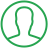# Python For Loop Index Range

Last Updated : Oct 6, 2023In this article we will show you the solution of python for loop index range, an index range for loop in Python allows you to iterate over a list of values instead of the actual values by using their indices.

When accessing both the index and the value of every element in a sequence or when performing an operation on each member in the sequence using its index, this is helpful.

The range() function, which produces an integer sequence from an initial value to an ending value (inclusive or exclusive) with an optional step size, can be used to generate the index range.

With an index range and a for loop, you can control how you iterate over a sequence and carry out intricate operations on its components.

Now move to the concept of python for loop index range.

## Step By Step Guide On Python For Loop Index Range :-

```import random
numbers = [random.randint(0, 100) for _ in range(10)]
print("List of random numbers:", numbers)
for i in range(len(numbers)):
if i == 0:
print("First number in the list is:", numbers[i])
elif i == len(numbers) - 1:
print("Last number in the list is:", numbers[i])
else:
print("Number at index", i, "is:", numbers[i])```
1. As you can see, we utilize the built-in random module in Python to create a list of 10 random numbers from 0 to 100. The code is written in for loop for the index range.
2. The random module, which offers a number of methods for generating random numbers and carrying out various random operations, is imported into the first line of the code.
3. We employ the function randint(), which produces a random integer between two predetermined integers.
4. Then, using the randint() function, we use a list comprehension to create a new list called numbers, which contains 10 randomly generated integers.
5. Then, we create a sequence of 10 integers using the range() function and a 10th argument, and we represent each one of these integers using the placeholder variable _.
6. Using the randint() method, we create a random integer between 0 and 100 for each integer in the sequence and add it to the numbers list.
7. The numbers list is then printed out along with a message stating that it is a list of random numbers.
8. Then, the for loop that comes after uses the range() function with an argument of len(numbers) to create a sequence of integers that correspond to the positions in the numbers list.
9. We utilise an if statement for each number in the sequence to determine whether the current index is the first, last, or any other index.
10. We print a message stating that the first integer in the list is represented by the first index if such is the case.
11. If it's the final index, a message stating that it's the final number in the list is printed.
12. If not, a message with the index and value of the current number is printed.

## Conclusion :-

As a result, we were able to understand the Python for loop index range.

We also learnt that one of the most effective ways to iterate over lists of values and execute operations on them using their indices in Python is to use a for loop with an index range.

You can become a more proficient and successful Python programmer by learning how to create index ranges using the range() function and how to modify a for loop to suit your needs.

I hope this article on python for loop index range helps you and the steps and method mentioned above are easy to follow and implement.

##About Pragati

Experienced coding content writer who enjoys breaking down complex concepts in programming languages like Java, Python, C, and C++. Over three years of experience producing interesting and relevant content for a variety of entities. Committed to providing concise and easy-to-understand articles that assist readers in easily understanding technology and industry trends in the world of coding and software development.